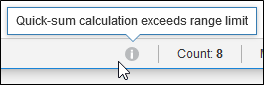1. Modeling
2. Working with Data
3. Quick Sum Bar

In a module or dashboard grid, when data is selected, simple automatic calculations are displayed in the Quick Sum Bar at the bottom of the screen.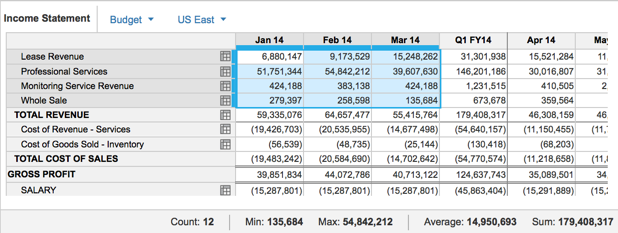The calculations displayed are Count, Min, Max, Average, and Sum. These calculations operate on number-formatted cells only, with the exception of the Average calculation, which includes the values of all the cells selected, regardless of format.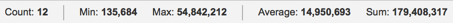• Count: the number of cells selected
• Min: the lowest value selected
• Max: the highest value selected
• Average: the average of all the values selected
• Sum: the total of all values selected

Empty cell values are not included in the calculations.

The format for the display of the calculations is the format of the first line item selected.

## Data Selections

The data selection can be:

• a range of contiguous cells;
• a column or row of data; or
• a nested selection.

When selecting data, all the cells highlighted, including summary cells, are included in the calculation. To ensure values aren’t included in quick sum calculations, hide rows or columns before making a selection.

Here, we can see quick sum values with summary values selected:

This shows the same grid, with the summary value hidden: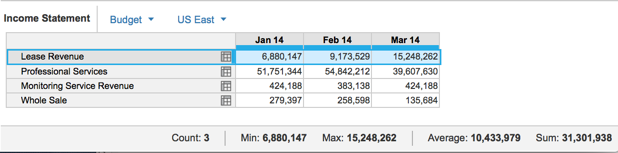## Show or hide the Quick Sum Bar

The Quick Sum Bar is shown by default but can be hidden by selecting Hide Quick Sum Bar from the ? menu in the global navigation bar. When it’s hidden, the option Show Quick Sum Bar is displayed.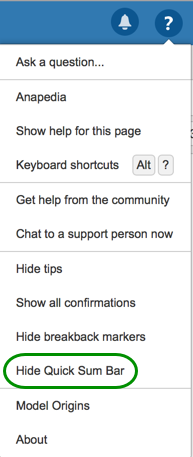## Tooltips

A number of tooltips provide information about why calculations are not completed.

### Number format

Values are calculated only for cells with the format Number. If cells with a different format are selected, a tooltip advises that multiple cell types have been selected.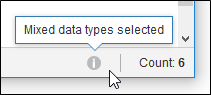In this situation, values will be calculated for cells with the Number format only.

### Data in memory

The calculations use values accessible in memory. If the data required to complete the calculation isn’t available in memory, a tooltip advises that there is insufficient data available to complete the calculation.### Limits of calculations

If the value of a calculation exceeds 9007199254740991 or -9007199254740991, a tooltip advises that the calculation exceeds the range limit and only the cell count will be displayed.